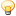##Newtons Method for Approximating Roots

Hi, i have this method here that approximates square roots. I was wondering what ppl thought about it and whether it is following a correct algorithm. It seems to work good and gave pretty close approximations for all my test casses. The only issue is on the very first call to the function, if 1 is passed in as a guess it returns bogus numbers.

Code:
```/*
* Newtons Method for Approx. square roots.
* Accepts 2 doubles.
* Argument 1 is the number to take the square root of.
* Argument 2 is the approximation/guess of the square root.
* x =  1/2( x + A/x)  <-- Formula being used.
*/
double approxSQRT(double A, double approx)
{
double previous=approx;

approx = (0.5)*(approx + ( A/approx));

//When sequential approximations have a negligable difference the
//   approximation should be very close to the actual square root.
if(previous - approx < 0.0000001 )
return(approx);

return( approxSQRT(A,approx));
}```